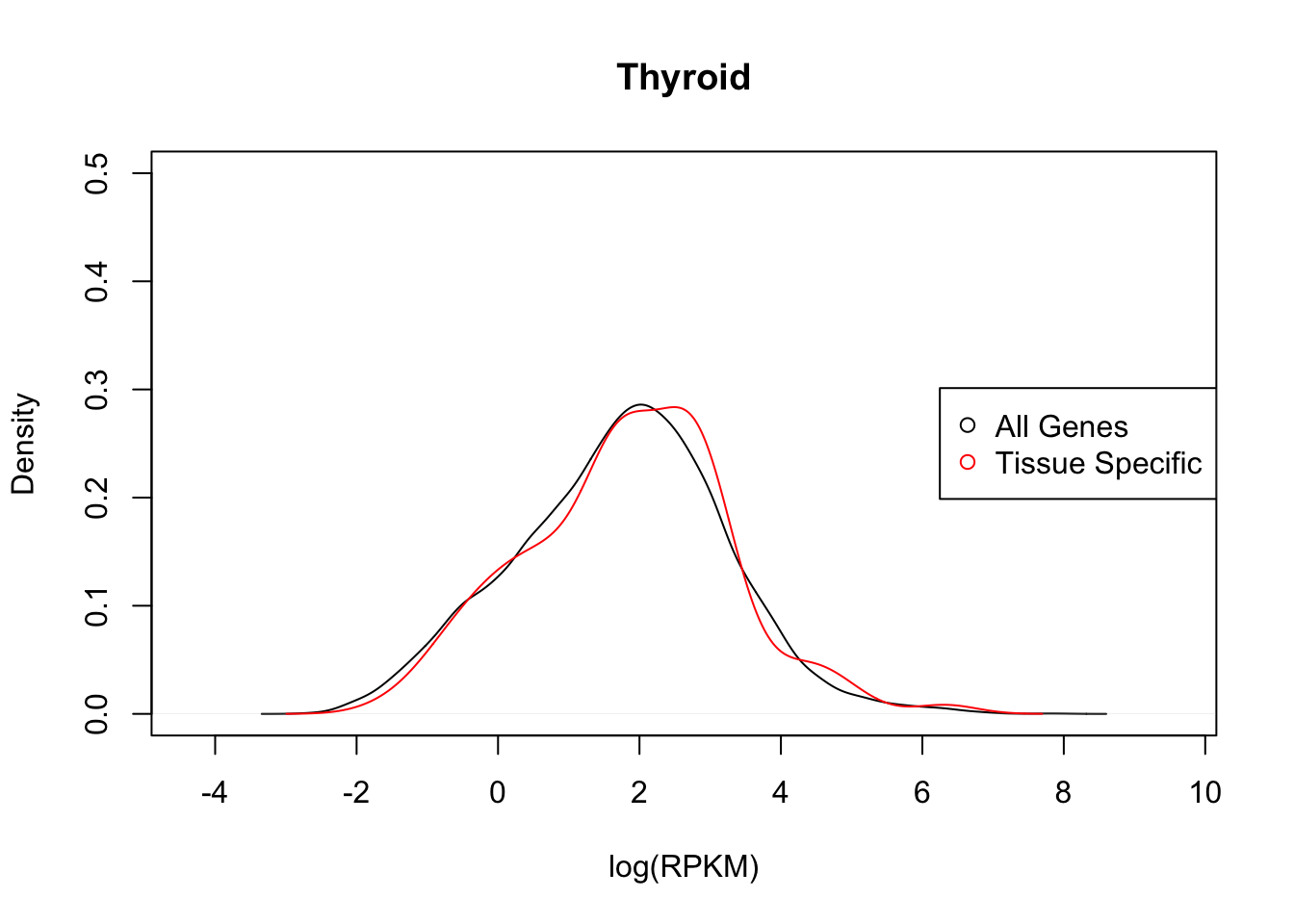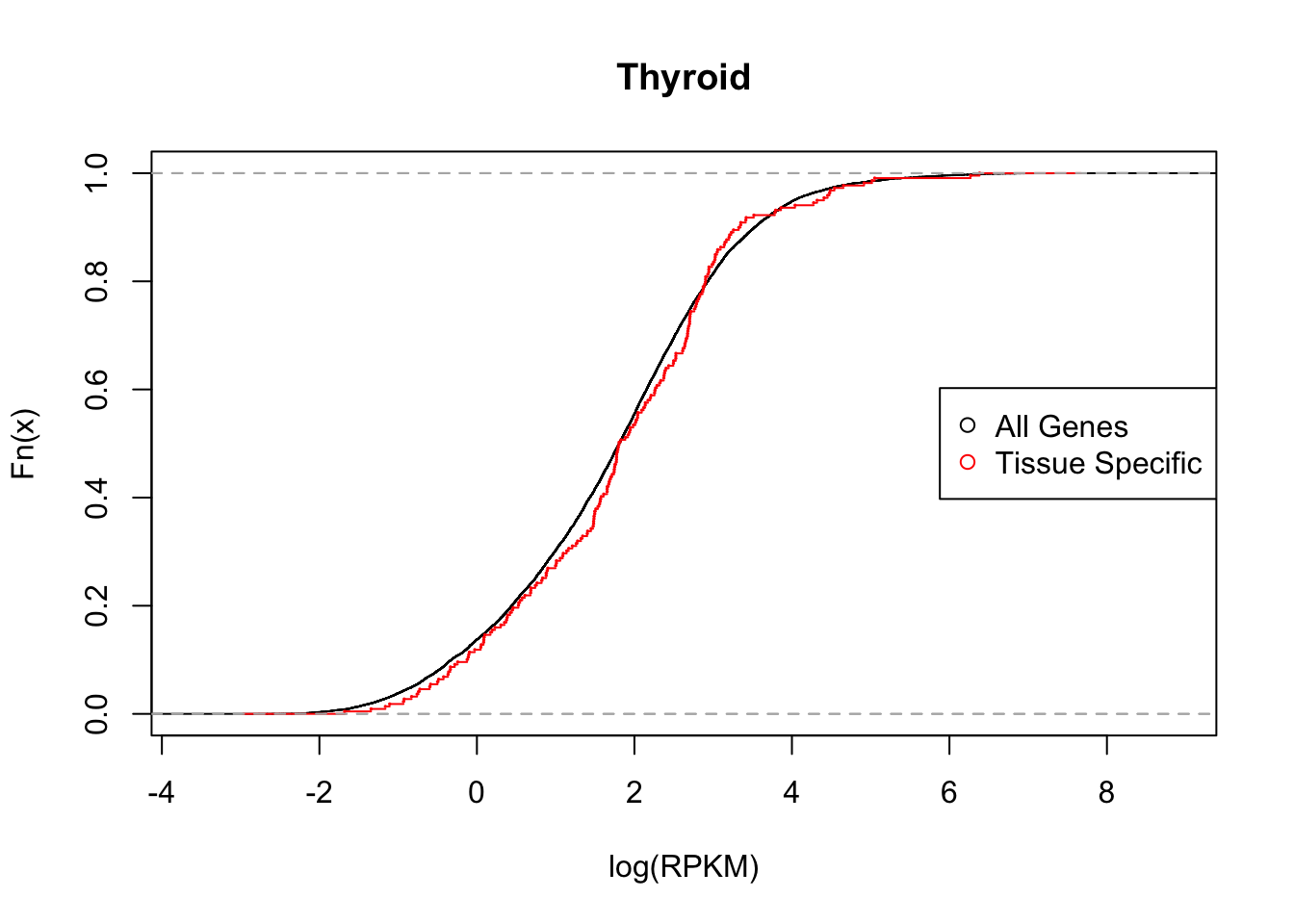Here, we simply plot the density of the average expression for all genes

Here we compare quantiles and plot data:

Thyroid:

``````#compare.distribution(tissuename = "Thyroid",lfsr = lfsr,curvedata = log(exp.sort),thresh = 0.05 )

plot_tissuespecifictwo(tissuename = "Thyroid",lfsr = lfsr,curvedata = log(exp.sort),title = "Test",thresh = 0.05,ymax=0.5 )````````plot_tissuespecificthree(tissuename = "Thyroid",lfsr = lfsr,curvedata = log(exp.sort),title = "Test",thresh = 0.05,ymax=1 )``Testis:

``````#compare.distribution(tissuename = "Testis",lfsr = lfsr,curvedata = log(exp.sort),thresh = 0.05)

plot_tissuespecifictwo(tissuename = "Testis",lfsr = lfsr,curvedata = log(exp.sort),title = "Test",thresh = 0.05 ,ymax=0.5)``````